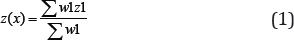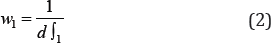info@biomedres.us   +1 (502) 904-2126   One Westbrook Corporate Center, Suite 300, Westchester, IL 60154, USA   Site Map

Impact Factor : 0.548

# Interpolation of Spatial Data Volume 9- Issue 1

Rae Zh Aliyev*

• Institute of Soil Science and Agrochemistry of NAS of Azerbaijan, Azerbaijan

Received: September 09, 2018;   Published: September 17, 2018

*Corresponding author: Rae Zh Aliyev, Institute of Soil Science and Agrochemistry of NAS of Azerbaijan, Azerbaijan

DOI: 10.26717/BJSTR.2018.09.001746

## Abstract

In the course of the study, we have disclosed methods for correcting and analyzing spatial data recorded in a vector format. The latter is best suited for spatial analysis of discrete objects. However, in the case when the spatial variable is represented as a field of scalar or vector magnitudes (for example, spatial concentration distribution of concentrations of heavy metals in soils or the velocity field of groundwater movement). Convenient ways of data recording is a raster format. This approach is most often used for phenomena of processes that are characterized by significant anisotropy. However, the characteristic feature of the inverse distance method is the fact that the interpolated value at the measured point is equal to the measured value.

## Introduction

### The Method of Spatial Data Interpolation

Figure 1 In it, the space consists of an infinite number of points and is divided into a certain number of rectangular fields called pixels, mudflows, and a specific value is attributed . Most often we own limited information about the spatial layout of the variable being studied. In general, our knowledge is reduced to a certain finite measuring points, on the basis of which conclusions are drawn about the possible distribution of quantities to the remaining points of space. Data collection at measuring points can be considered as a random series, based on which the forecasting of parameters outside the testing points is performed. This process is called interpolation of spatial data. There are many methods of interpolating data. Below are the selected methods that are available in the AreGIS program. One of the most popular ways of interpolating spatial data is the Inverse Distance Weighted method.This is the method, the main one of which is the autocorrelation of spatial data . Autocorrelation means that the magnitude of the spatial variable at the point x is related to the values near this point, and the binding force decreases together with the distance d. The value of the variable under study at the interpolation point z (x) is defined as the weighted average of the points measured around z, and the force of its influence on the nearest points w, decreases simultaneously with the distance to the measured point d.There are several methods for determining the values of the coefficients of the force of influence. The most frequently used fraction of the square of the distance:This method minimizes the influence of measurement points located farther than the value z (x) calculated at the interpolation point, since a double increase in distance leads to a fourfold decrease in the force of influence. This corresponds to the position of decreasing the strength of spatial autocorrelation at the same time as the distance increases. Interpolation algorithms based on the method of reciprocal distances make it possible to search for measurement points in the radius of the interpolation point, as well as to determine the points from which the values will be taken for calculations [3,4]. This is possible, since for d, tending to zero, the quantity w, is directed to infinity. This property of the method is an indisputable plus, but (paradoxically!) Simultaneously and its greatest limitation, which gave results in the example of creating a soil-numeric map of the Zakatala region of Azerbaijan (Figure 2).

This means that the extremum of the predicted spatial distribution is always assigned to the measuring points. If we consider the measured points. If we consider the values obtained at measuring points as a random series, then the probability that the testing point corresponds to the localization of the largest or smallest value in the analyzed field is close to zero.

### Creating A Raster of Spatial Distribution of the Values of a Given Parameter

Interpolation of spatial data in the ArcMap program is possible thanks to the application Spatial Analyst. Therefore, the first task will be the appropriate adaptation of the working area.

a) In the Tools menu, select Extensions.

b) In the Extensions window, select the selection box near the Spatial Analyst position and click Close.

c) Thus, the tool for spatial analysis of raster and vector data was activated. Now the functionality of the interface is expanded with a new toolbar.

d) Right-click on the empty space in the toolbar.

e) In the menu, select Spatial Analyst. The toolbar will be added to the image.

The technique of spatial data interpolation will be considered using the example of creating a soil contamination map for cesium in the territory of X. For this, two vector layers are necessary:

a) polygonal - with the boundaries of the study area,

b) point-to-point with the results of measurements of cesium concentrations at the study sites.

### We Begin the Analysis by Selecting the Parameters of the Spatial Analyst Application

a) In the Spatial Analyst menu on the toolbar, select Options.

b) In the Options window, go to the General tab.

c) In the Working directory column, we denote the path to the folder where the raster files created as a result of the analysis will be written.

d) In the Analysis mask, define the territory for analysis using the shape file, that is, we select the prepared polygonal layer with the boundaries of the investigated territory.

e) In the Analysis Coordinate System frame, select a coordinate system for raster's with analysis results (for example, Pulkovo 1942).

f) Go to the Extent tab. Here we indicate the spatial boundaries of the analysis (the coordinates of the extreme points). Select the Same as Layer option (that is, the prepared layer with the boundaries of the study area) from the Analysis Extent list.

g) Now go to the Cell Size tab to determine the value of the effective raster. From the list choose the option As Specified below, in the Cell Size column enter 250. This means that the layers created will calculate the concentration of cesium in the soil. In the columns below, the number of rows and columns will be displayed for the desired resolution of the analysis.

h) Confirm the changes-OK.

I. In the Spatial Analyst menu, select Interpolate to Raster, and then inverse Distance weighted.

II. In the Input Points column, select the file with the primary data for interpolation (Punkty).

III. The Z value field is used to select the attribute to be interpolated. From the list of available attributes, select "Cesium".

IV. In the Power column, specify the distance to calculate the magnitude of the force of influence points. We recommend leaving the automatic value of this parameter.

V. The pointer of the graph Search radius type determines the way of selecting points for interpolation. Choose the Variable. Due to this, it will be possible to determine the number of measuring points involved in the prediction and their maximum distance from the interpolation points.

VI. In the Search Radius Settings box, enter the value in the Number of Points-column [5-8]. The graph of the maximum distance is left empty. In this way, it is achieved that interpolation at each point will be based on the 5 nearest measuring points.

VII. Note that the Cell Size column is automatically assigned a value of 250, respectively, with previously executed commands.

VIII. In the graph we enter the arbitrary name of the effective raster layer, for example, Cesium.

IX. Select OK to start the interpolation process. If the data interpolation process expires correctly, the result in the form of a raster layer will be added to the work area [9,10].

### The Automatically Raster Layer Opens in Classified Mode

This means that the values will automatically be classified based on the 9-step scale with the corresponding colors. However, you can change the layer display mode. To do this, go to the window with the properties of the layer (similarly as for vector layers).

a) Go to the Symbology tab where two layers are displayed for selection: Classified (Separate) and Stretched (solid). Choose the second.

b) Using the Color Ramp list, select the color scheme for the layer. Here the color scale is represented in the form of an increase in the intensity of the color. If we want to show a change in the intensity of the color in the reverse order, then select Invert. In the Label (inscription), you can specify the values that will be in the map legend.

c) Go to the Stretch frame. From the Type list, select the value of Standard Deviation. In the graph n we introduce the value 4.

d) Confirm the changes-OK.

In this case, the spatial distribution of the variable is shown in a continuous way, which allows us to better analyze the spatial variability of the characteristic under study. However, for greater readability, the user of the map must be able to directly determine the value of the variable at a given point.1

a) Go to the window with the properties of the layer and select the Display tab.

b) Select the Show Mar Tips option and click OK.

c) In the image window of the map, draw the cursor over the area of the raster layer. Near the cursor, the value of the variable at that point will be highlighted.

The above method gives good results if we work with the card in the cipher version. However, when the created card must be paper, the interactive mode with the digital card becomes impossible. In this case, to better display the spatial variability of the parameter, you should apply an isoline map, which can be superimposed on the raster layer.

a) In the Spatial Analyst menu, select Surface Analysis, then Coytrour.

b) In the open Contour Input Surface window, the name of the created raster layer should appear. Otherwise you must select the appropriate layer from the file list.

c) In the Contour definition box, you can define the contour section and the base contour. In the Contour interval column, enter 0.5 and leave the value in the Base case. In the box below, information will appear on the choice of parameters, namely, the number of contours and extreme values associated with isolines.

d) In the Out Features column, specify the localization and the name of the source file.

e) Click OK. To the image will be added a vector layer with drawn on the basis of the raster layer isolines and assign them inscriptions.

f) Go to the window with the properties of the Izolinie Cez layer and select the Label tab.

g) Denote the Label features in this layer.

h) In the Label Field from the list, select Contour.

i) Set the size and color of the font of the inscriptions. To change the way the labels are placed, you can change the different options that will be available after selecting Placement Properties.

j) Confirm the changes, OK.

k) For a final increase in the readability of the image, you can add a layer with the test points and sign them with the appropriate concentrations of cesium in the soil (similar to the layer) Izolinie-Cez).